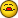# Help with Vlookup and MAX functions

I

#### Iriemon

I'm trying to set up a formula to find the MAX value in a range using
VLOOKUP. All I get is a "#value" error with the formula below. I tried to
substitute "MAX('Future Phase in values'!I22" for the column number but it
doesn't like it.

Any help will be greatly appreciated. I know there must be a simpler way. I
can always put in a "helper" column that will find the Max value and then
have the VLOOKUP return that column but I'm trying to avoid adding any
additional columns to my "Future Phase in values" worksheet. Can it be done
using VLOOKUP or do I need to use another function?

Thanks

=VLOOKUP(A3968,'Future Phase in values'!A23429,MAX('Future Phase in
values'!I22,FALSE))

J

#### JP Ronse

Hi,

Try with closing the MAX function

=VLOOKUP(A3968,'Future Phase in values'!A23429,MAX('Future Phase
invalues'!I22),FALSE)

Wkr,

JP

E

#### Excel Curious

Try

=VLOOKUP(A3968,'Future Phase in
values'!A23429,8+(MATCH(MAX(I39683968),I39683968,0)),FALSE)

Let me know if it works

I

#### Iriemon

The formula below doesn't work properly, I think it's the

8+(MATCH(MAX(I39683968),I39683968,0))

that is not functioning.

A little better explaination :

Item number is in cell A3968 on sheet1.
Pricing data is on worksheet "Future Phase in values"

Once it finds the item number on "Future phase in values, return the MAX
value from columns "I" through "P"

"Future phase in values" has data from row 2 through row 3429

Thanks for the suggestions, I'm still trying to get this worked out.

E

#### Excel Curious

Ok...

=VLOOKUP(A3968,'Future Phase in
values'!A23429,8+MATCH(MAX(INDIRECT("'Future Phase in
values'!I"&MATCH(A3968,'Future Phase in
values'!A2:A3429,0)+1&""&MATCH(A3968,'Future Phase in
values'!A2:A3429,0)+1)),INDIRECT("'Future Phase in
values'!I"&MATCH(A3968,'Future Phase in
values'!A2:A3429,0)+1&""&MATCH(A3968,'Future Phase in
values'!A2:A3429,0)+1),0),FALSE)

There may be a more "streamline" way of doing this, but this is what I could
come up with.